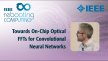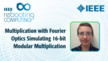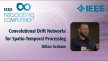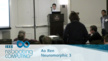# Convolution

View this topic in
In mathematics and, in particular, functional analysis, convolution is a mathematical operation on two functions f and g, producing a third function that is typically viewed as a modified version of one of the original functions. (Wikipedia.org)

# 22,249 resources related to Convolution

### Conferences related to Convolution

2020 IEEE International Instrumentation and Measurement Technology Conference (I2MTC)

The Conference focuses on all aspects of instrumentation and measurement science andtechnology research development and applications. The list of program topics includes but isnot limited to: Measurement Science & Education, Measurement Systems, Measurement DataAcquisition, Measurements of Physical Quantities, and Measurement Applications.

Oceans 2020 MTS/IEEE GULF COAST

To promote awareness, understanding, advancement and application of ocean engineering and marine technology. This includes all aspects of science, engineering, and technology that address research, development, and operations pertaining to all bodies of water. This includes the creation of new capabilities and technologies from concept design through prototypes, testing, and operational systems to sense, explore, understand, develop, use, and responsibly manage natural resources.

2019 41st Annual International Conference of the IEEE Engineering in Medicine & Biology Society (EMBC)

The conference program will consist of plenary lectures, symposia, workshops andinvitedsessions of the latest significant findings and developments in all the major fields ofbiomedical engineering.Submitted papers will be peer reviewed. Accepted high quality paperswill be presented in oral and postersessions, will appear in the Conference Proceedings and willbe indexed in PubMed/MEDLINE & IEEE Xplore

2019 IEEE Computer Society Annual Symposium on VLSI (ISVLSI)

This Symposium explores emerging trends and novel ideas and concepts in the area of VLSI. The Symposium covers a range of topics: from VLSI circuits, systems and design methods to system level design and system-on-chip issues, to bringing VLSI experience to new areas and technologies. Future design methodologies as well as new CAD tools to support them will also be the key topics. ISVLSI 2019 highlights a special theme of Neuromoprhic Computing. Over almost two decades the Symposium has been a unique forum promoting multidisciplinary research and new visionary approaches in the area of VLSI.

2019 IEEE International Conference on Image Processing (ICIP)

The International Conference on Image Processing (ICIP), sponsored by the IEEE SignalProcessing Society, is the premier forum for the presentation of technological advances andresearch results in the fields of theoretical, experimental, and applied image and videoprocessing. ICIP 2019, the 26th in the series that has been held annually since 1994, bringstogether leading engineers and scientists in image and video processing from around the world.

### Periodicals related to Convolution

The IEEE Aerospace and Electronic Systems Magazine publishes articles concerned with the various aspects of systems for space, air, ocean, or ground environments.

Experimental and theoretical advances in antennas including design and development, and in the propagation of electromagnetic waves including scattering, diffraction and interaction with continuous media; and applications pertinent to antennas and propagation, such as remote sensing, applied optics, and millimeter and submillimeter wave techniques.

Speech analysis, synthesis, coding speech recognition, speaker recognition, language modeling, speech production and perception, speech enhancement. In audio, transducers, room acoustics, active sound control, human audition, analysis/synthesis/coding of music, and consumer audio. (8) (IEEE Guide for Authors) The scope for the proposed transactions includes SPEECH PROCESSING - Transmission and storage of Speech signals; speech coding; speech enhancement and noise reduction; ...

The theory, design and application of Control Systems. It shall encompass components, and the integration of these components, as are necessary for the construction of such systems. The word `systems' as used herein shall be interpreted to include physical, biological, organizational and other entities and combinations thereof, which can be represented through a mathematical symbolism. The Field of Interest: shall ...

Broad coverage of concepts and methods of the physical and engineering sciences applied in biology and medicine, ranging from formalized mathematical theory through experimental science and technological development to practical clinical applications.

### Xplore Articles related to Convolution

IEEE Access, None

With the popular application of direct part mark (DPM) technology, DPM code inspection has been a hot issue in the machine vision. It mainly consists of two steps, namely, localization and decoding. DPM code localization is a key and complex step in the DPM code inspection. However, the traditional localization methods suffer from complex imaging environment, involving various imaging background, ...

2014 11th International Conference on Electrical Engineering/Electronics, Computer, Telecommunications and Information Technology (ECTI-CON), 2014

In Digital Signal Processing, the convolution and deconvolution with a very long sequence is ubiquitous in many application areas. The basic blocks in convolution and de-convolution implementation are multiplier and divider. They consume much of time. This paper presents a direct method of computing the discrete linear convolution, circular convolution and deconvolution. The approach is easy to learn because of ...

2018 17th International Symposium on Distributed Computing and Applications for Business Engineering and Science (DCABES), 2018

In this paper, we proposed the concept of mask convolution, which operates on irregular shaped foreground, and could gain improvement on the margin of the object. In particular, we prove that this operation could be done in an efficient way. The extra calculation than normal convolution only lies on mask image convolution, and one time elements-wise multiply and division. To ...

IEEE Photonics Technology Letters, 2009

A concise formulation of the frequency-dependent finite-difference time-domain (FDTD) method is presented using the trapezoidal recursive convolution (TRC) technique for the analysis of a Drude-Lorentz model. The TRC technique requires single convolution integral in the formulation as in the recursive convolution (RC) technique, while maintaining the accuracy comparable to the piecewise linear RC (PLRC) technique with two convolution integrals. The ...

IEEE Transactions on Circuits and Systems II: Express Briefs, 2006

Novel one- and two-dimensional systolic structures are designed for computation of circular convolution using distributed arithmetic (DA). The proposed structures involve significantly less memory and less area-delay complexity compared with the existing DA-based structures for circular convolution. Besides, it is shown that the proposed systolic designs for circular convolution can be used for computation of linear convolution as well

### Educational Resources on Convolution

#### IEEE.tv VideosTowards On-Chip Optical FFTs for Convolutional Neural Networks - IEEE Rebooting Computing 2017Multiplication with Fourier Optics Simulating 16-bit Modular Multiplication - Abigail Timmel - ICRC 2018Convolutional Drift Networks for Spatio-Temporal Processing: IEEE Rebooting Computing 2017Designing Reconfigurable Large-Scale Deep Learning Systems Using Stochastic Computing - Ao Ren: 2016 International Conference on Rebooting Computing

#### IEEE-USA E-Books

• With the popular application of direct part mark (DPM) technology, DPM code inspection has been a hot issue in the machine vision. It mainly consists of two steps, namely, localization and decoding. DPM code localization is a key and complex step in the DPM code inspection. However, the traditional localization methods suffer from complex imaging environment, involving various imaging background, illumination, imaging distance, and exposures. Furthermore, the target itself, i.e., the DPM code, could be severely polluted or worn. Aiming at improving the performance and robustness of DPM code localization, an efficient method with depthwise separable convolution is proposed in this paper. The optimized network model has the advantages of few parameters, high computational efficiency, high precision localization, and good generalization ability. Meanwhile, the precision of DPM code region is improved with the help of multi-scale prediction. The experiments on our DPM code localization database demonstrate the effectiveness and flexibility of the proposed method in comparison with the YOLOv3 network and the Tiny_YOLO network. Furthermore, the proposed method can estimate the exposure level of the DPM code region, which is benefiting to the DPM code recognition and enables the adaptive ability.

• In Digital Signal Processing, the convolution and deconvolution with a very long sequence is ubiquitous in many application areas. The basic blocks in convolution and de-convolution implementation are multiplier and divider. They consume much of time. This paper presents a direct method of computing the discrete linear convolution, circular convolution and deconvolution. The approach is easy to learn because of the similarities to computing the multiplication of two numbers. The most significant aspect of the proposed method is the development of a multiplier and divider architecture based on Ancient Indian Vedic Mathematics sutras Urdhvatriyagbhyam and Nikhilam algorithm. The results show that the implementation of linear convolution and circular convolution using vedic mathematics is efficient in terms of area and speed compared to their implementation using conventional multiplier & divider architectures. The coding is done in VHDL. Simulation and Synthesis are performed using Xilinx ISE design suit 14.2. Simulated results for proposed 4×4 bit Vedic convolution circuit shows a reduction in delay of 88% than the conventional method and 41% than the OLA method.

• In this paper, we proposed the concept of mask convolution, which operates on irregular shaped foreground, and could gain improvement on the margin of the object. In particular, we prove that this operation could be done in an efficient way. The extra calculation than normal convolution only lies on mask image convolution, and one time elements-wise multiply and division. To verify the necessity of mask convolution, we use mean filtering on retina images to eliminate spots. Compared to normal convolution, the proposed method could obtain clearer image on the margin of foreground, which is beneficial for subsequent CNN-based vessel segmentation model. We also proved that this method can be extended to other convolution kernels such as Gaussian and sharpen filters.

• A concise formulation of the frequency-dependent finite-difference time-domain (FDTD) method is presented using the trapezoidal recursive convolution (TRC) technique for the analysis of a Drude-Lorentz model. The TRC technique requires single convolution integral in the formulation as in the recursive convolution (RC) technique, while maintaining the accuracy comparable to the piecewise linear RC (PLRC) technique with two convolution integrals. The TRC technique is introduced not only to the traditional explicit FDTD, but also to the unconditionally stable implicit FDTD based on the locally one-dimensional (LOD) scheme. Through the analysis of a surface plasmon waveguide, the effectiveness of the TRC technique is investigated for both explicit FDTD and LOD-FDTD, along with the existing RC and PLRC techniques.

• Novel one- and two-dimensional systolic structures are designed for computation of circular convolution using distributed arithmetic (DA). The proposed structures involve significantly less memory and less area-delay complexity compared with the existing DA-based structures for circular convolution. Besides, it is shown that the proposed systolic designs for circular convolution can be used for computation of linear convolution as well

• A high performance digital architecture for computing 2D convolution utilizing the quadrant symmetry of the kernels is proposed in this paper. Pixels in the four quadrants of the kernel region with respect to an image pixel are considered simultaneously for computing the partial products of the convolution sum. A novel data handling strategy to identify the pixels to be fed to different processing elements helps reducing the data storage requirements in the circuitry. The new design results in 75% reduction in multipliers and 50% reduction in adders when compared with the conventional systolic architecture. The proposed architecture design is capable of performing convolution operations with 14/spl times/14 kernel at a rate of 57 1024/spl times/1024 frames per second in a Xilinx 's Virtex 2v2000ff896-4 FPGA.

• In the generalized-version of modern, MIMO discrete-time control, known as Discrete/Continuous (D/C) Control Theory, the traditional matric convolution integral B in the traditional, exact, discrete-time state-model: x((k +1)T) = Ax(kT)+ Bu(kT) is replaced by a more general convolution-matrix BH that has two independent kernels, which evolve in counterflow directions. The numerical-evaluation of the generalized matric convolution-integral BH is essential in practical applications of D/C-type discrete-time control, but the dual-kernel, counterflow nature of the matric convolution integral BH complicates the application of traditional numerical methods for evaluating convolution integrals. In this paper, symbolic software (MAPLE) is used to develop the exact, closed-form, explicit analytical expressions for the matric convolution-integral BH(T) for a family of simple, time- invariant, low-order examples. Those explicit, closed-form analytical results provide much needed "truth-models" against which numerical-evaluations of BH , using various alternative numerical-integration schemes, can be confidently compared.

• In signal processing systems, the fundamental computation is nothing but convolution which is found in many application areas. The main building blocks are the multipliers to calculate convolution. But multipliers are the main power consuming elements. In recent years power is an important constraint. Now days, linear convolution has been implemented using distinct types of multipliers to decrease power dissipation. In this paper, modulo linear convolution have been proposed using modulo multipliers based on Radix-8 booth encoding algorithm for bits n=8, 16, 32 and 64. The modulo design is coded in Verilog HDL, Xilinx 14.7 has been used to perform Simulation and Synthesis. The proposed technique efficiently speeds up the computation which in turn decreases power dissipation, hardware resources and area significantly.

• This paper proposes a hybrid wavelet convolution network (HWCN) which is composed of a scattering convolution component and a convolution neural component. The hierarchical end-to-end network implements sparse-coding and high-dimensional reconstruction for inverse problem through cascade convolutions. With the pre-defined scattering convolutions from nonlinear operators, the network can be tailored in accordance with the frequency property to provide sparse code candidates, and the convolution neural component could automatically select and weight these candidates for sparse coding. Given a tiny dataset, HWCN could train complex deep network with better generalization by regularization from scattering convolutions, and thereby is a competitive alternative to convolutional neural networks (CNN). Moreover, we further demonstrate that HWCN is a superior selection of sparse- coding based image super-resolution and achieves state-of-the-art performance.

• Recently the previously reported Modified Fermat Number Transform (MFNT) based on Right Circular Convolution (RCC) was extended to form a Quadratic MFNT (QMFNT) by introducing Left-angle Circular Convolution (LCC) and interpreting the combined result as a quadratic representation of the resulting convolution output. This paper introduces a new Modified Discrete Fourier Transform (MDFT) that relies on a similar combination of RCC and LCC. The MDFT enables overlap- add FFT block processing to be implemented without zero padding, resulting in reduced computational complexity and potentially reduced power requirements in nanoscale VLSI implementations.

### Standards related to Convolution

No standards are currently tagged "Convolution"